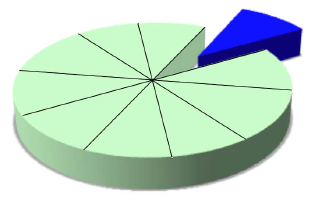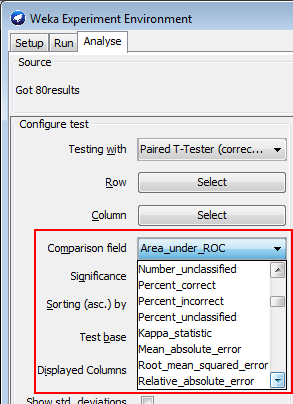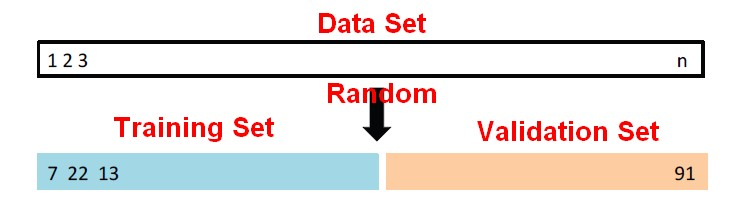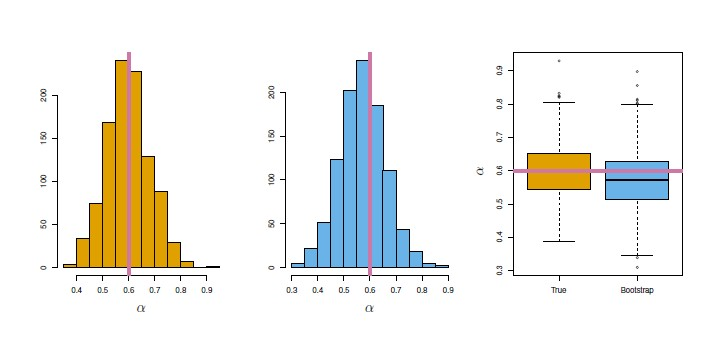# Model Building - ReSampling Validation

Resampling method are a class of methods that estimate the test error by holding out a subset of the training set from the fitting process, and then applying the statistical learning method to those held out observations.

For supervised Learning problems, separate data sets are required for building (training) and testing the predictive models.

The training error is too optimistic. The more we fit to the data, the lower the training error but the test error can get higher if we over fit and it often will. For this reaons, models are fitted on part of the data, and then evaluated against on a holdout set (Test set).

Generally, the Build Activity splits the data into two mutually exclusive subsets:

• the training set for building the model: Evaluate the model on the training set. Performance estimates obtained on the training set are overly optimistic because of overfitting.
• the test set for testing the model. Evaluate the model on a separate test set

However, if the data is already split into Build and Test subsets, you can run a Build activity and specify the Split step.

Of course, the build data (training data) and test data must have the same column structure.

## Methods

Practical rule of thumb:

Resampling methods:

• Cross-validation is a very important tool to get a good idea of the test set error of a model.
• Bootstrap, on the other hand, is most useful to get an idea of the variability or standard deviation of an estimate and its bias.

### Two-fold Validation

Two-fold validation: randomly divide the available set of samples into two parts: a training set and a validation or hold-out set.

### Percentage Split

Percentage Split (Fixed or Holdout) Leave out random N% of the data. For example, you might select 60% of the rows for building the model and 40% for testing the model. The algorithm is trained against the trained data and the accuracy is calculated on the whole data set.

### Cross-validation

Also known as validation:

### Bootstrap

Related: Bootstrap: Generate new training sets by sampling with replacement

Bootstrap is a very clever device for using the one, single training sample you have to estimate things like standard deviations.

Recommended Pages(Statistics|Data Mining) - (K-Fold) Cross-validation (rotation estimation)

Cross-validation, sometimes called rotation estimation is a resampling validation technique for assessing how the results of a statistical analysis will generalize to an independent new data set. This...Data Mining - (Test|Expected|Generalization) Error

Test error is the prediction error that we incur on new data. The test error is actually how well we'll do on future data the model hasn't seen. The test error is the average error that results from using...Data Mining - (Parameters | Model) (Accuracy | Precision | Fit | Performance) Metrics

Accuracy is a evaluation metrics on how a model perform. rare event detection Hypothesis testing: t-statistic and p-value. The p value and t statistic measure how strong is the...Data Mining - Test Set

The test set is a set that is used to validate the model. Test set represent the foresight (unknown data, real data) whereas training Set represents the hindsight. Generally, the test data is created...Data Mining - Training (Data|Set)

In statistics, the training data is the sample whereas in data mining, machine learning, the training data is often a subset of the data set. See Training Set represents the hindsight whereas test set...Machine Learning - (Overfitting|Overtraining|Robust|Generalization) (Underfitting)

A learning algorithm is said to overfit if it is: more accurate in fitting known data (ie training data) (hindsight) but less accurate in predicting new data (ie test data) (foresight) Ie the model...R - Logistic Regression

logistic regression in R We have a call to GLM where we gives: the direction: the response, and the predictors, the family equals binomial. This parameter tells GLM to fit a logistic regression...Statistical Learning - Two-fold validation

Two-fold validation is a resampling method. It randomly divides the available set of samples into two parts: a training set and a validation or hold-out set. The model is fit on the training set,...Statistics - (Case-control|retrospective) sampling

Statistics - (Case-control|retrospective) sampling The case-control sampling comes in a study in which subjects arenot randomized to exposed or unexposed groups, but rather the subjects are observed...Statistics - Bootstrap Resampling

Bootstrap is a powerful resampling method for assessing uncertainty in estimates and is particularly good for getting their: standard errors and confidence limits. Why is the bootstrap useful? The...